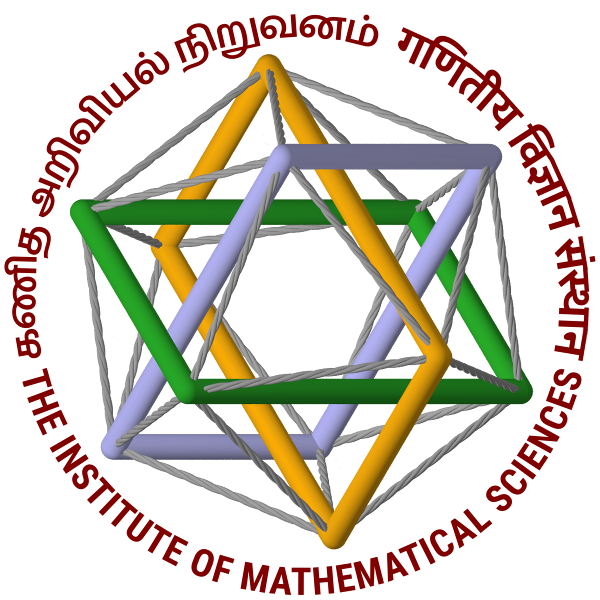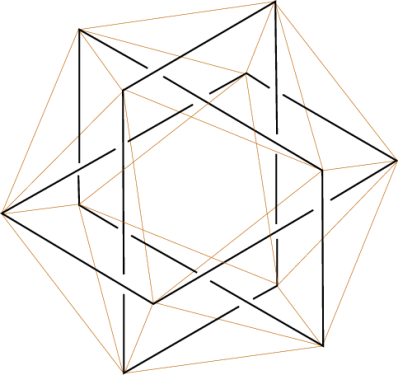# IMSc Logo

The design for a logo for the Institute of Mathematical Sciences (IMSc) was conceived and designed by IMSc faculty members: Parameswaran Sankaran, T. R. Govindarajan and Kapil Paranjape.### Philosophy

At the time, IMSc had three major subject disciplines Physics, Mathematics and Computer Science. These areas are interlinked and yet separate. The fundamental idea is that the Borromean rings present such a relationship between three entities. Since all the areas are pursued in their theoretical/abstract guise at IMSc, it is also natural that the logo be abstractly definable rather than merely drawn, sketched or photographed. At the same time it should be physically realisable and the abstract definition should be capable of being presented as an algorithm. Finally, it would be nice if other numerological accidents were present to enhance the beauty, applicability and intricacy of the logo.

Subsequently, a fourth group has been established at IMSc (Computational Biology) but the logo has been retained.

The physical description

The logo consists of a collection of three “golden” rectangles made out of tubing which extrudes along as the orthogonal plane sections of an icosahedron. Eight equilateral triangles made of “rope” lie in the eight octants and join the appropriate corners of the rectangles. The shape outlined is a regular isocahedron. The letters “IMSc” will ﬂoat at the centre of this shape.

### Ruler and Compass construction

The planar version of this logo can be constructed using ruler and compass as explained below. We begin by constructing a regular hexagon with side of length a. We also construct the length b = a ⋅ (√5 - 1) which is the side of a golden rectangle with longer side of length a; this construction is part of the construction of a regular pentagon. Let X, Y and Z be the diagonals of the regular hexagon. Let X- and X+ be segments of length b which are parallel to X and lie with their centres at the two ends of Z. Similarly, place Y - and Y + which are of length b and parallel to Y at the ends of X, and again Z- and Z+ which are of length b and parallel to Z and lie at theends of Y . The end points of these last six lines are the corners of the projection of the icosahedron.

So let Z± (respectively X± and Y ±be the lines parallel to Z (repsectively X and Y ) which join the appropriate endpoints of X± (respecitvely Y ± and Z±. The parallelogram bounded by X± and Z± (repsectively bounded by Y ± and X±, and bounded by Z± and Y ±) is the planar projection of a rectangle in the XZ plane (repsectively Y X plane and ZY plane). These three rectangles are linked in the topological type of borromean rings. Moreover, each corner of each rectangle lies in exactly one quadrant of the corresponding plane. The coordinate planes divide space into eight octants, each of which is bounded by three quadrants. We can thus join the three corners that lie in each octant to get the eight triangles.

### Some other aspects

As is well known the group of symmetries of the icosahedron has been extensively studied by Felix Klein. It is isomorphic to the group of projective automorphisms of a projective line over a ﬁelds with 5 elements. This group is one of the few ﬁnite groups that occurs within the special orthogonal group in three dimensions; through the relation between the latter group and the special unitary group in two complex dimensions, there has been some interest in the icosahedral group from theoretical physicists.... notably string theorists. The crystallographic nature of this group has also attract theoretical chemists.

The above representation of the Borromean Rings is known to have inspired the scultor John Robinson to make a real scuplture very similar to the one above. This ﬁgure is known to have enthused geometer H. S. M. Coxeter as well. Borromean rings and the surrounding inter-linkage have resulted in their extensive use in all kinds of geometrical art and a number of other logos (but none of the logos seem to use this particular representation!).

There is a lot of literature (and mysticism!) surrounding the golden rectangle at its relation to the Fibonnacci sequence. It is also the simplest example of the Chakravala (or continued fraction) solution of the quadratic equation x2 - Dy2 = 1 (with D = 1).

The icosahedron is also closely related to the singularity x3 + y5 = z12 + z22 + .... + zn2. John Milnor showed that the link of this singularity is an “exotic” sphere for n = 2; it is homeomorphic to but not diffeomorphic to a 7-dimensional sphere.